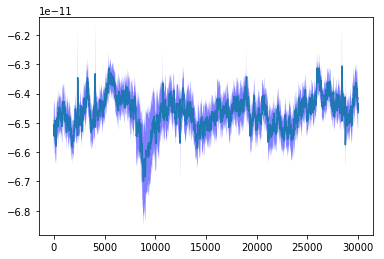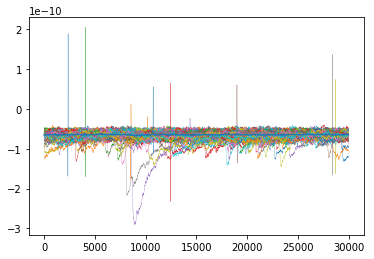In :
# -*- coding: utf-8 -*-
"""
Created on Thu May 16 16:20:55 2019

@author: Healey  (Luke Remage-Healey)
"""
import numpy as np
import matplotlib.pyplot as plt
import os
from neo import io

"""takes a stack of Igor PMPulse files ('ibw') inside a folder, reads them in, and
generates an overlay as well as mean+sem graph for quick visualization,
and and array 'arry.npy' to be used later for analysis"""

Out:
"takes a stack of Igor PMPulse files ('ibw') inside a folder, reads them in, and\ngenerates an overlay as well as mean+sem graph for quick visualization, \nand and array 'arry.npy' to be used later for analysis"
In :
def addfolder():
"""helper function to add '.ibw' files from a designated folder and feed them iteratively below"""
textfiles = []
files = os.listdir()
for i in range(len(files)):
if files[i].endswith('.ibw'):
textfiles.append(files[i])

return textfiles


In :
"""generates an order of the files in the folder, in case this is needed later"""
order = []
for i in folder:
order.append(i)

In :
"""reads the Igor files using the neo IO, and converts them to Analogsignals.
See neo documentation for more on this format"""

a = []
for i in folder:
r = io.IgorIO(filename=i)

return a

In :
"""below runs the routine and converts to a numpy array. The numpy 'reshape'  converts
3D arrays into 2D arrays, to allow them to be saved, manipulated, etc.
There are probably better ways of doing this, but this works."""

arr1 = np.array(arr2)

arr = arr1.reshape(len(arr1), len(arr1))

In :
arr.size

Out:
5400000
In :
"""just to check it is working"""

Out:
'just to check it is working'
In :
#once done adding the trials, you can then plot the average:

u = np.mean(arr, axis=0).reshape(arr.size)
x = np.array([[list(range(u.size))]])
std = np.std(arr, axis=0).reshape(arr.size)
se = (np.std(arr, axis=0)/np.sqrt(len(arr))).reshape(arr.size)
plt.figure('inward currents')
plt.plot(u)
plt.fill_between(list(range(u.size)), (u+se), (u-se), facecolor='blue', alpha=0.5)
plt.show()In :
# to get the overlays of all plots:

fig, ax = plt.subplots()

for i in range(len(arr)):

ax.plot(arr[i], linewidth=0.3, markersize=0.005, label = str(i))

ax.plot()
#    ax.legend()

#to add the mean+-se to the plot:

ax.plot(u, label = 'mean + sem')
ax.fill_between(list(range(u.size)), (u+se), (u-se), facecolor='blue', alpha=0.5)

Out:
<matplotlib.collections.PolyCollection at 0x9bf7fc23c8>In :
print("VOILA!")

VOILA!

In [ ]: